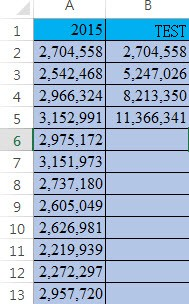#0

## PHP Array 累加!!!

Dear 前輩們~

``````		\$my_array=null;
foreach (\$result["IT_TEST"] as \$AAA) {

\$my_array[]=\$AAA;

}
print '<pre>';
print_r (\$my_array);
print '<pre>';
``````louischou iT邦新手 4 級 ‧ 2017-08-04 18:34:03 檢舉
\$my_array[]=\$AAA;
\$my_array2[]+=\$AAA;

### 2 個回答

1

``````\$my_array=null;
\$my_array_sum=null;//累加用
\$result["IT_TEST"]=array(2704558,2542468,2966324,3152991);

foreach (\$result["IT_TEST"] as \$AAA) {
\$my_array[]=\$AAA;
\$my_array_sum[]= ( \$my_array_sum==null?\$AAA:(\$my_array_sum[(count(\$my_array_sum)-1)])+\$AAA );
//將上一個累加結果+目前的值

}

print '<pre>';
print_r (\$my_array);
print_r (\$my_array_sum);//累加結果
print '<pre>';
``````

``````\$my_array=[];
\$my_array_sum=[];
``````

``````foreach (\$result["IT_TEST"] as \$value) {
\$my_array[] = \$value;
\$my_array_sum[] = array_sum(\$my_array);
}
``````

(我把table也秀出來了)

``````<?php
\$data = [ 2704558 , 2542468 , 2966324 , 3152991 ];
\$results = [];
\$sum = 0;

foreach(\$data as \$value){

\$sum += \$value;

\$results[] = [
'value' => \$value ,
'sum' => \$sum
];

}
?>

<table>
<tr>
<th>value</th>
<th>sum</th>
</tr>
<?php foreach(\$results as \$item):?>
<tr>
<td><?=\$item['value']?></td>
<td><?=\$item['sum']?></td>
</tr>
<?php endforeach?>
</table>
``````

``````\$my_array=[];
\$my_array_sum=[];
``````

(同時也考量到官方維護的問題。)

fillano iT邦超人 1 級 ‧ 2017-08-07 09:56:07 檢舉

``````\$from = array(1, 2, 3);
\$to = array_reduce(\$from, function(\$pre, \$cur){ return \$pre + \$cur; }, 0);
echo \$to;//6
``````

@費大公 code有錯字XD

(通常打錯字會改，但我打錯字笑出來就不想改了。)

fillano iT邦超人 1 級 ‧ 2017-08-07 11:24:44 檢舉

fillano iT邦超人 1 級 ‧ 2017-08-07 11:29:59 檢舉

0
``````\$my_array=[];
foreach(\$result["IT_TEST"] as \$i => \$v) {
\$my_array[\$i] = \$my_array[\$i-1] + \$v;
}
print '<pre>';
print_r (\$my_array);
print '<pre>';
``````

\$my_array[ -1 ] ???

wiseguy iT邦超人 1 級 ‧ 2017-08-07 17:59:12 檢舉

`\$my_array[\$i] = \$i ? \$my_array[\$i-1] + \$v : \$v;`